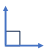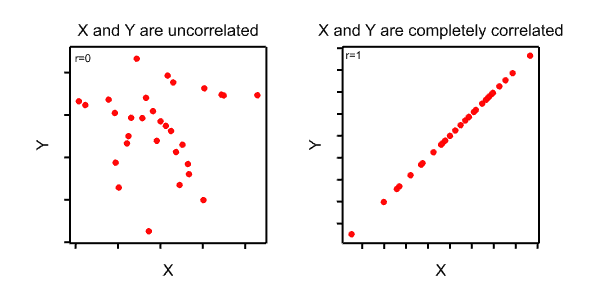What are Balance, Efficiency and Independence?

### Home### Blogs# Experimental Design: What are Balance, Efficiency and Independence?

Dr. Ruth Butler

15 November 2023There are several concepts relating to the design of experiments that were developed in the pre-computer and early computer age, to facilitate the design and then analysis of trials. This was especially important pre-computers, when only hand calculating machines were available. These days, new methods and modern computers can be used to analyse data from even the most difficult of designs. However, the concepts are still relevant today: For example, a design with general balance means the data can be analysed with Analysis of Variance, a simpler and more interpretable analysis than is often the case for designs without this property.

A few of these concepts are discussed below.Independent, Orthogonal, Aliased, Confounded

These four terms are strongly related to each other.

Independent: Two variables (or factors) are independent if neither variable contains any information about the other. For example, if you have two factors A and B, with all combinations of the levels of these factors, and equal replication, then there is no information about factor B related to factor A, and vice versa. Similarly, if two variables X and Y are completely un-correlated (r=0), there is no information about Y in X. If you carried out a regression of Y on X, the estimated slope would be 0, and the constant would be the mean of Y.Orthogonal: If two variables (or factors) are independent, they are also ‘orthogonal’. The term is derived from ‘orthogonal’ meaning at ‘right-angles’, which in turn relates to the angle between two plotted vectors being 90°.

Confounding and Aliasing:

Chris Brien describes these in an excellent way in an email to the Genstat list from March 2023:

“Confounding was used by Fisher to describe the mixing up of treatment contrasts with block contrasts and aliasing was used by Finney to describe the mixing up of treatment contrasts with other treatments contrasts.”

Here ‘mixing up’ essentially means the terms cannot be estimated independently of each other. Chris goes on to say:

“However, there is a third kind of mixing of information that occurs in designed experiments and that is marginality, as discussed by Nelder, who also called it intrinsic aliasing. Marginality is a property of terms in a model and occurs irrespective of the replications of the levels of the factors involved in the terms”

‘Marginality’ means that a term is a sub-term of another term. For example, the main effect of A is marginal to the interaction of A with B (A.B). What this means is that if you include the interaction A.B in your analysis before the main effect, then there is nothing left relating to the main effect- it has been absorbed into the interaction. (Except… Type III sums of squares somehow enables this in a way that ANOVA experts like John Nelder disapprove of.)

#### Strata

For a trial that has more than one level of information (such as Blocks and Plots within Blocks), then these levels are called strata (singular: stratum). Genstat’s ANOVA makes defining and interpreting the strata very easy, with the ANOVA laid out to easily show them:

Table 1: Strata for four simple designs, with TREATMENTSRUCTURE A*B. A and B each have 2 levels. Twenty plots for Five replicates, except for the Latin square where there are 16 plots and 4 replicates.

#### Efficiency

The efficiency of a particular design relates to the variance (or precision) of a comparison between individual treatment pairs. The variance can be calculated beforehand, as a function of the unknown random variation (error mean square) s2. The variance is compared with that for the same comparison for a randomized block or completely randomized design. An efficiency of 1 (the maximum) means the comparison is fully efficient. If there is more than one treatment factor, then efficiencies can be calculated for pairwise comparisons for the levels of each factor and terms (like the interaction A.B) in the model. If a factor appears in more than one strata in the analysis, then you can get an efficiency for that factor within each strata. For most standard designs, the pairwise efficiency is the same for all pairs. However, for more complex designs, such as those that can be generated with CycDesigN, the efficiencies can vary between treatment pairs. Thus, CycDesigN uses an ‘Average efficiency’, which is an average of the efficiencies for all treatment pairs. (The average used is the harmonic mean).

#### Balance

‘Balance’ has more than one definition. Some definitions describe ‘balance’ as meaning ‘equally replicated’. However, treatment sets can be balanced even with unequal replication: In relation to treatment factors, two factors are balanced if they are orthogonal.

Balance also relates to Blocking structures. Again, the requirement is orthogonality between the blocking factors.

Finally, ‘General Balance’ (Payne & Tobias) requires that the Treatment factors are orthogonal, that the blocking factors are orthogonal, AND (approximate description) that the treatment factors have equal efficiency within each strata where they are estimated. The mathematical definition of General Balance requires some complex matrix and vector concepts, but it is not necessary to understand these. General Balance essentially means that you have an efficient design where the various treatment and block terms can be estimated independently of each other. The easiest way to check whether a design has general balance is to run a ‘dummy’ ANOVA in Genstat. To do this, work out what the Block and Treatment structures are. Then set these up in Genstat (using the BLOCKSTRUCTURE and TREATMENTSTRUCTURE commands). Then, follow this with the ANOVA command with no parameters. For example, if you have Blocks and Plots within Blocks, the Blocking structure would be “Block/Plot”, and if your treatments are a factorial combination of A and B, the Treatments structure would be A*B. Therefore, in Genstat, you would use the following commands:

BLOCK Block/Plot

TREAT A*B

ANOVA

### Reference

Payne, R.W. & Tobias, R.D. 1992. General balance, combination of information and the analysis of covariance. Scandinavian journal of statistics, 19, 3-23.